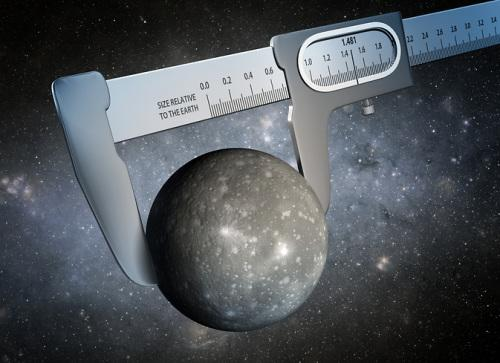EN

### Introduction to the Knowledge of Dimension Measurement Errors

share:

2021/05/05

As long as there are measurements, there must be measurement results, which will inevitably produce errors, which will affect the measurement accuracy. Therefore, we should have a comprehensive and systematic understanding of the characteristics, nature and classification of errors, and finally find out reasonable and scientific ways to eliminate them. It is particularly important in precision dimension measurement. So let's first clarify the terms of measurement.

Interpretation of measurement-related concepts

Error: The difference between the test results and the true values of the measurements.

Repeatability: Test the divergence of the results repeatedly under the same test conditions.

Reproducibility: Consistency between repeated test results under varying test conditions.

True Value: The value of a measurement under ideal test conditions. (Truth does not exist in reality)What is measurement error?

In the process of direct measurement, because of the inaccuracy of measuring tools and the imperfection of measuring methods, the measurement results are inaccurate and deviate from the true value, which is the error. In indirect measurement, because of the errors of the direct measurement results, the errors can be transmitted to the final results, and can also make it deviate from the true value.

Because the truth value (also known as theoretical value) can not be accurately obtained, in fact, the agreed truth value is used. The agreed truth value needs to be expressed by the uncertainty of measurement, so the measurement error can not be accurately obtained.

Uncertainty of measurement: It shows that the dispersion of the measured value is reasonably assigned. It is related to people's understanding of the measured value. It is an interval obtained by analysis and evaluation.

The measurement error is the difference that indicates that the measurement result deviates from the true value. It exists objectively but people can not determine it.

For example, the measurement results may be very close to the true value (i.e., the error is very small), but due to insufficient understanding, the value given by people falls in a larger area (i.e., the measurement uncertainty is larger); or in fact, the measurement error may be larger, but due to insufficient analysis and estimation, the uncertainty given is smaller. Therefore, when evaluating measurement uncertainty, various factors should be fully considered, and the evaluation of uncertainty should be verified.

As mentioned above, it is known that errors exist in all measurements, so it is necessary to discuss errors and understand their regularity, nature, source and size. The analysis of experimental errors is of great significance for people to improve experiments, improve their precision and accuracy (the significance of precision and accuracy will be discussed later), and even new discoveries.

Errors are divided into random errors and systematic errors.

Systematic error: The measurement error caused by the inherent error of the measuring tool (or instrument), the defect of the measuring principle or the theory of the measuring method itself, the experimental operation and the restriction of the psychological and physiological conditions of the experimenters themselves is called systematic error.

The characteristic of system error is that under the same measurement condition, the result of repeated measurement is always too large or too small, and the value of the error varies with a certain rule. The method of reducing system error can usually change the measuring tool or method, and can also consider the correction value of the measurement result.

Random error: Random error is also called accidental error. Even under the ideal condition of completely eliminating system error, repeated measurement of the same measurement object will still produce measurement error due to various accidental and unpredictable uncertainties, which is called random error.

Random error is characterized by repeated measurements of the same measurement object. The errors of the measured results show irregular fluctuations, which may be positive (the measurement results are too large) or negative (the measurement results are too small), and the fluctuations of the absolute value of errors are irregular. However, the distribution of errors obeys the statistical law, showing the following three. Features: unimodality, that is, the error is smaller than the error is larger; symmetry, that is, the probability of positive error and negative error is equal; boundedness, that is, the probability of large error is almost zero.

The Relation among Errors, Random Errors and System Errors

From the definition of error, random error and system error, we can see that:

Error = measurement result - true value

= (Measurements - Total Means) + (Total Means - True Values)

= Random Error + System Error

Measurement results = true value + error = true value + random error + system error

According to the distribution law of random error, increasing the number of measurements and processing the measurement results according to statistical theory can reduce the random error.

Related concepts: What is repetition accuracy?

If the random error of measurement value is small, that is, the fluctuation of each measurement result is small, the measurement repeatability is good. It is called good precision or good stability. Therefore, the magnitude of accidental error reflects the precision of measurement.

The above content describes the related concepts in dimension measurement, and I believe you have a new understanding of dimension measurement instruments.

### TECHNICAL SOLUTION

MORE+

You may also be interested in the following information### Automated Identification And Detection Solution On Earphone Module Chip Bubble Defects### Characters And Soldering Tin Defects Recognition Detection For Hexahedrons Inductor Or Resistor### Detect Out The Scratches and Fingerprint On Bank Card### FREE CONSULTING SERVICE